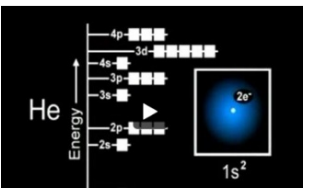# Problem: Part A. In the animation, you can see that the electrons occupy different orbitals according to the energy level of each orbital. A single box represents an orbital. The unpaired electron is represented as ↿ whereas the paired electrons in the same orbital are represented by two arrows pointing in opposite directions: ↿⇂ . Watch the animation and identify which of the following statements are correct.A) The He atom has two electrons that have parallel spin in its 1s orbital.B) The C atom has two unpaired electrons. C) In the Li atom, the 3s, 3p, and 3d orbitals have different energies.D) The arrangement of the orbitals is the same in a multielectron atom and a single-electron atom. E) The He atom has one electron each in 1s and 2s orbitals.F) Electrons generally occupy the lowest energy orbital first.The electron configuration of an atom describes how the electrons are distributed among the various orbitals of the atom. Watch the animation to learn about the rules that are useful when writing the electron configuration of elements.

###### FREE Expert Solution
80% (120 ratings)View Complete Written Solution
###### Problem Details

Part A. In the animation, you can see that the electrons occupy different orbitals according to the energy level of each orbital. A single box represents an orbital. The unpaired electron is represented as ↿ whereas the paired electrons in the same orbital are represented by two arrows pointing in opposite directions: ↿⇂ . Watch the animation and identify which of the following statements are correct.

A) The He atom has two electrons that have parallel spin in its 1s orbital.
B) The C atom has two unpaired electrons.

C) In the Li atom, the 3s, 3p, and 3d orbitals have different energies.
D) The arrangement of the orbitals is the same in a multielectron atom and a single-electron atom.

E) The He atom has one electron each in 1s and 2s orbitals.
F) Electrons generally occupy the lowest energy orbital first.

The electron configuration of an atom describes how the electrons are distributed among the various orbitals of the atom. Watch the animation to learn about the rules that are useful when writing the electron configuration of elements.